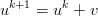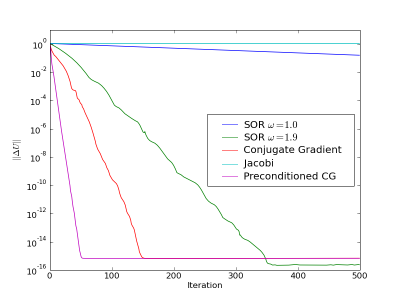## Monday, March 30, 2009

### Ares/Orion Slip and Classic Mistake

The latest news about the new NASA Constellation program indicates that they are having cost and schedule problems, this is no surprise, everyone has cost and schedule problems. The surprising thing is that they are deleting tests from the planned program development.

CxP attempted to protect the schedule and budgetary pressures by offsetting these additional strains by deleting test items - notably on the Upper Stage. However, this only proved to cause further disconnects throughout the program.

I suppose it should not be too surprising, this is a classic program management mistake. When the schedule gets tight and the costs get too high above estimates, cut testing. Why? Because it is at the end of the program, and program managers have a bit of hubris that leads them to think that the design is sound because they worked so hard on it up-front. Which is obviously the case because they had to spend more time than they thought in that stage of the program, right? Maybe, but also maybe not, that's why the tests are critically important: to validate the design performance. No slide-show, CAD drawing, CFD or FEA simulation can provide that sort of knowledge.

I can't understand why someone would think that under-testing a new product that is probably delayed and expensive because of its new technology and complexity would produce a good result. Rather than removing unneeded technology or complexity, rather than simplifying or reducing the requirements, they are rushing to field a fragile system. It is unfortunate that the burden of this foolishness is borne by the astronaut corps and not the program managers responsible for these short-sited expediencies. The program manager will probably get a reward, move to a new job, and then astronauts will be at undue risk in an already risky business.

## Monday, March 16, 2009

### Complex Step Jacobian-Free Newton-Krylov

Newton's method are really useful for solving non-linear systems.Each iteration of the Newton's method requires solving a linear equation:where the Jacobian is the matrix of partial derivativesand then applying the update to the solution vector:For small systems it is tractable to analytically calculate the Jacobian and invert it directly. This rapidly becomes intractable for realistic engineering problems because the number of unknowns can be on the order of 1e6 (if you have a good workstation) to 1e9 (if you have a big cluster). Rather than explicitly creating a 1e6-squared matrix and inverting it at every iteration, an iterative method that only requires the action of the matrix on a vector can be used (such as conjugate gradient or GMRES). Then we can approximate this matrix vector product bywhich is a good improvement in storage over the matrix approach, but we can improve it still further by using a complex stepThis means we only need one system evaluation to approximate the Jacobian, and much more accurate estimates can be made. As shown in a previous post it is second order accurate, and not as limited by subtractive cancellation as the standard difference approach is.

Here's a simple example of the idea in Maxima

/* trying out complex step finite differences for jacobian free newton
krylov methods */

/* define a simple system of equations */
F : zeromatrix(2,1);
F[1,1] : x*x + 2*x;
F[2,1] : 2*x + x*x;

/* analytic Jacobian */
J : zeromatrix(2,2);
J[1,1] : diff(F[1,1],x);
J[1,2] : diff(F[1,1],x);
J[2,1] : diff(F[2,1],x);
J[2,2] : diff(F[2,1],x);

u : zeromatrix(2,1);
v : zeromatrix(2,1);
u[1,1] : 1.5;
u[2,1] : 1.0;
v[1,1] : 0.2;
v[2,1] : 0.1;

c_step : 0.0 + 1.0e-200*%i; /* complex step */

/* approximate Jacobian using complex step */
Jv_approx : (
ev(F, x = u[1,1] + c_step*v[1,1], x = u[2,1] + c_step*v[2,1])
) / imagpart(c_step);
imagpart(Jv_approx);

/* evaluate the analytic Jacobian to compare */
Jv_analyt : ev(J.v,x=u[1,1],x=u[2,1]);

A good survey article introducing the JFNK method and describing some applications:
Jacobian-free Newton-Krylov methods: a survey of approaches and applications

Nice short paper about using complex steps in finite differences:
Using Complex Variables to Estimate Derivatives of Real Functions

## Sunday, March 1, 2009

### Three Dimensional Poisson's Equation

This is a follow up to the post I wrote a while back about implementing numerical methods. The goal is to demonstrate a work-flow using tools that come included in the Fedora Linux (and many other) distributions.
1. In Maxima
• Define governing equations
• Substitute finite differenceexpressions for differential expressions in the governing equations
• Output appropriate difference expressions to Fortranusing f90()
2. In Fortran: wrap the expression generated by Maxima in appropriate functions, subroutines and modules

3. In Python
• Compile modules with f2py, which comes packaged along with numpy
• Write the high-level application code, parsing input decks, performing optimization, grid convergence studies, or visualizations that use the compiled Fortran modules

### Maxima

The governing equation is the three-dimensional Poisson's equation.In Cartesian coordinatesThe Maxima code to define this equation is straightforward:
depends(u,x); depends(u,y); depends(u,z); /* Poisson's equation: */ p : 'diff(u,x,2) + 'diff(u,y,2) + 'diff(u,z,2) = -f;
Now that we have the continuous differential equation defined we can decide on what sort of discrete approximation we are going to solve. The simple thing to do with second derivatives is to use central differences of second order accuracy, which require only information from closest neighbours in the finite difference grid. Replacing the differential expressions with difference expressions is accomplished in Maxima with the ratsubst() function.
/* substitue difference expressions for differential ones: */
p : ratsubst((u[i-1,j,k] - 2*u[i,j,k] + u[i+1,j,k])/dx**2 , 'diff(u,x,2), p);
p : ratsubst((u[i,j-1,k] - 2*u[i,j,k] + u[i,j+1,k])/dy**2 , 'diff(u,y,2), p);
p : ratsubst((u[i,j,k-1] - 2*u[i,j,k] + u[i,j,k+1])/dz**2 , 'diff(u,z,2), p);
/* substitute the correct array value of f:*/
p : ratsubst(f[i,j,k], f, p);
This assumes that the solution u and the forcing function f are stored in three dimensional arrays, if not then the indexing in the difference expressions becomes more complicated. If we want to apply a Gauss-Seidel method to solve our elliptic problem we just need to solve p for u[i,j,k], and then dump that expression to Fortran format.
gs : solve(p, u[i,j,k]);
del : part(gs,2) - u[i,j,k];
/* output for fortran: */
with_stdout("gs.f90", f90(d = expand(del)));
This gives the expression for the update to the solution at each iteration of the solver.

### Fortran

The Maxima expressions developed above need control flow added so they get applied properly to our solution array.
do k = 1, nz
do j = 1, ny
do i = 1, nx
d = (dy**2*dz**2*u(i+1,j,k)+dx**2*dz**2*u(i,j+1,k)+dx**2*dy**2* & u(i,j,k+1)+dx**2*dy**2*dz**2*f(i,j,k)+dx**2*dy**2* & u(i,j,k-1)+dx**2*dz**2*u(i,j-1,k)+dy**2*dz**2*u(i-1,j,k))/ & ((2*dy**2+2*dx**2)*dz**2+2*dx**2*dy**2)-u(i,j,k) u(i,j,k) = u(i,j,k) + w*d
end do
end do
end do
The expression for the update was generated from our governing equations in Maxima, one of the things to notice is the  w  that multiplies our update, this is so we can do successive over relaxation. Also, we'll go ahead and package several of these iterative schemes into a Fortran module
module iter_schemes
implicit none
contains

...bunches of related subroutines and functions ...

end module iter_schemes

### Python

Now we want to be able to call our Fortran solution schemes from Python. Using F2py makes this quite simple:
[command-line]\$ f2py -m iter_schemes -c iter_schemes.f90
This should result in a python module iter_schemes.so that can be imported just as any other module.
import numpy
from iter_schemes import *

... do some cool stuff with our new module in Python ...

### Conclusion

This approach might seem like over-kill for the fairly simple scalar equation we used, but think about the complicated update expressions that can be generated for large vector partial differential equations like the Navier-Stokesor Maxwell's equations. Having these expressions automatically generated and ready to be wrapped in loops can save lots of initial development and subsequent debugging time. It also makes generating unit tests easier, and so encourages good development practice. But does it work? Of course, here's the convergence of the Gauss-Seidel and SOR schemes discussed above along with a Jacobi scheme and a conjugate gradient scheme applied to a 50 by 50 by 50 grid with a manufactured solution.The plot was generated with the Python module matplotlib.